# Improper Integrals

 Exam 2 Wed Mar 1: 7pm-7:50pm in ?? Today: 7.8 Improper Integrals Monday - president's day holiday (and almost my bday) Next -- 11.1 sequences

Example 5.7.1   Make sense of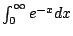. The integrals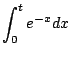make sense for each real number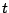. So consider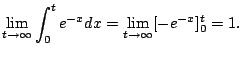Geometrically the area under the whole curve is the limit of the areas for finite values of.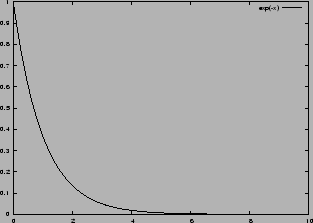Example 5.7.2   Consider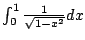(see Figure 5.7.2).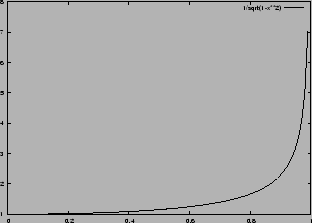Problem: The denominator of the integrand tends to 0 as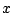approaches the upper endpoint. Define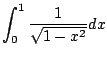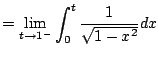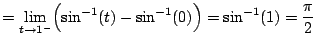Here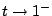means the limit astends to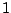from the left.

Example 5.7.3   There can be multiple points at which the integral is improper. For example, consider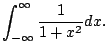A crucial point is that we take the limit for the left and right endpoints independently. We use the point 0 (for convenience only!) to break the integral in half.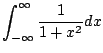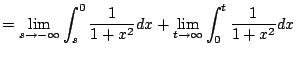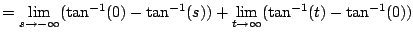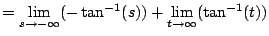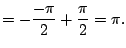The graph of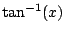is in Figure 5.7.3.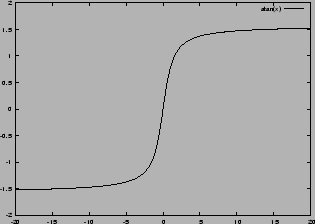Example 5.7.4   Brian Conrad's paper on impossibility theorems for elementary integration begins: The Central Limit Theorem in probability theory assigns a special significance to the cumulative area function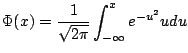under the Gaussian bell curve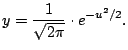It is known that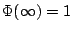.''

What does this last statement mean? It means that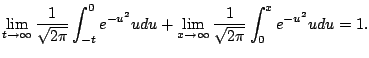Example 5.7.5   Consider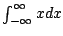. Notice that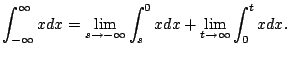This diverges since each factor diverges independtly. But notice that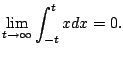This is not whatmeans (in this course - in a later course it could be interpreted this way)! This illustrates the importance of treating each bad point separately (since Example 5.7.3) doesn't.

Example 5.7.6   Consider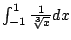. We have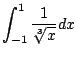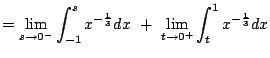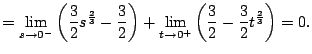This illustrates how to be careful and break the function up into two pieces when there is a discontinuity.

 NOTES for 2006-02-22 Midterm 2: Wednesday, March 1, 2006, at 7pm in Pepper Canyon 109 Today: 7.8: Comparison of Improper integrals 11.1: Sequences Next 11.2 Series

Example 5.7.7   Compute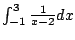. A few weeks ago you might have done this: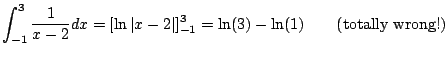This is not valid because the function we are integrating has a pole at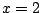(see Figure 5.7.4). The integral is improper, and is only defined if both the following limits exists: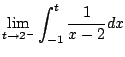and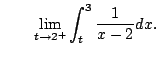However, the limits diverge, e.g.,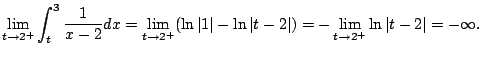Thusis divergent.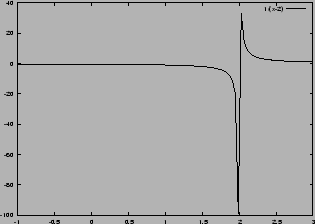Subsections
William Stein 2006-03-15